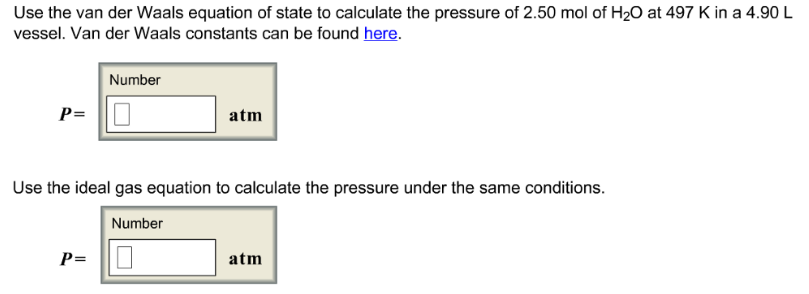# Problem: Use the van der Waals equation of state to calculate the pressure of 2.50 mol of H2O at 497 K in a 4.90 L vessel. Van der Waals constants can be found here. Use the ideal gas equation to calculate the pressure under the same conditions.

###### FREE Expert Solution
86% (163 ratings)###### Problem Details

Use the van der Waals equation of state to calculate the pressure of 2.50 mol of H2O at 497 K in a 4.90 L vessel. Van der Waals constants can be found here.

Use the ideal gas equation to calculate the pressure under the same conditions.